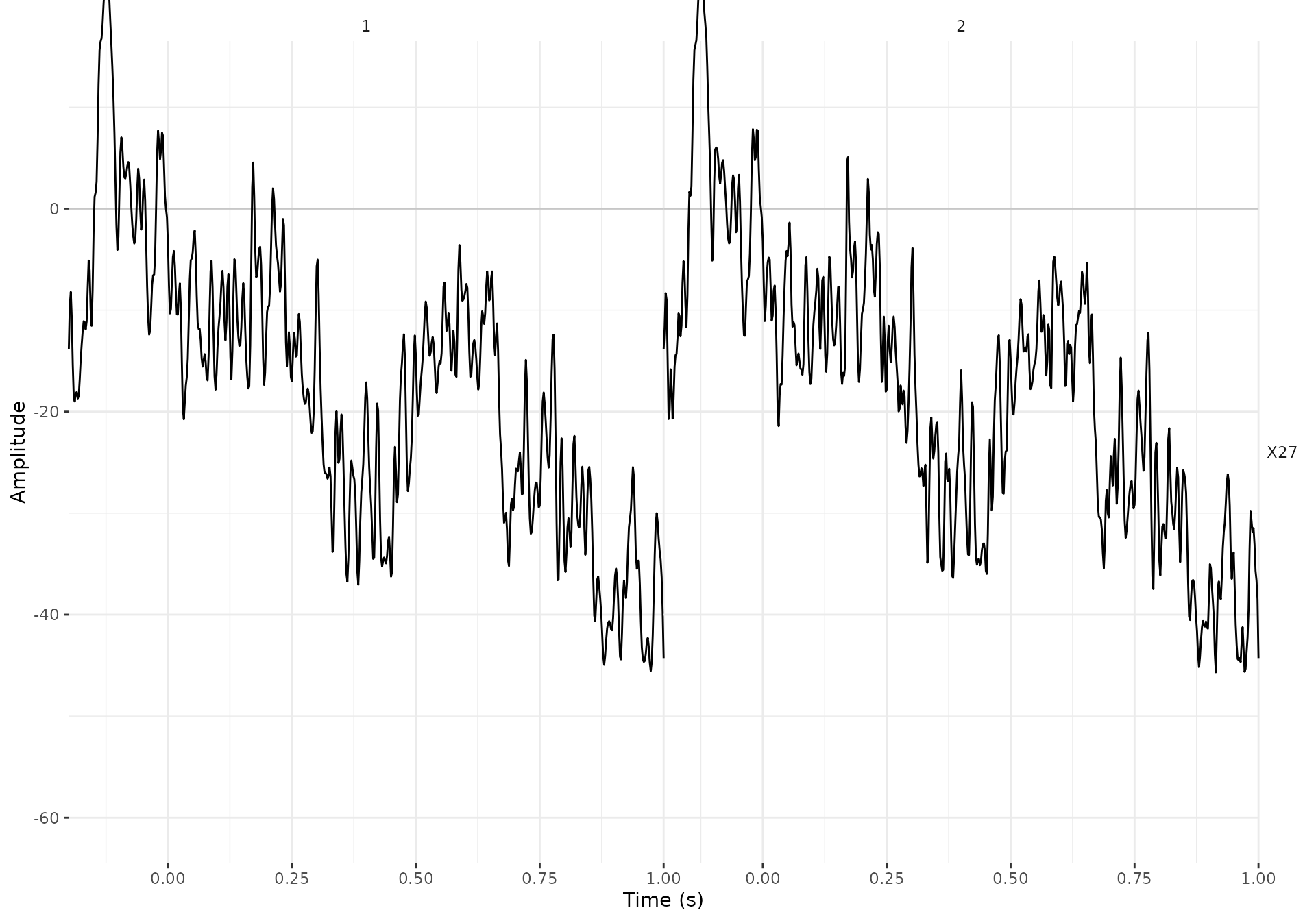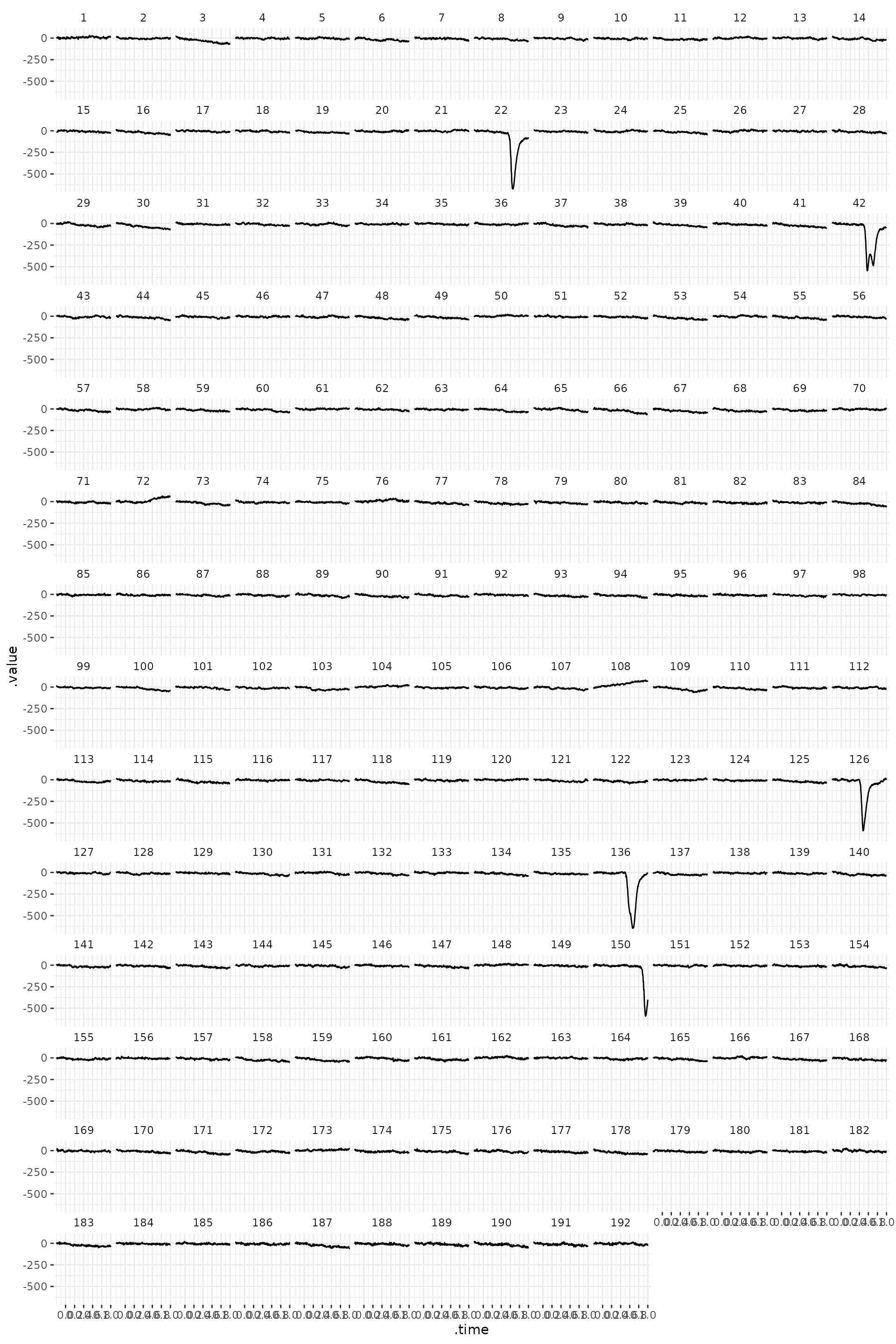,

This tutorial is an adaptation (and some parts are a verbatim copy) of Fieldtrip’s Preprocessing - Reading continuous EEG data. Fieldtrip is a great MATLAB toolbox for MEG and EEG analysis. Here I show, how we would do a very similar analysis with eeguana. (No previous experience with Fieldtrip is needed to follow this vignette).

The preprocessing of data refers to the reading of the data, segmenting the data around interesting events such as triggers, temporal filtering, and optionally re-referencing.

There are largely two alternative approaches for preprocessing, which especially differ in the amount of memory required (and processing time). The first approach is to read all data from the file(s) into memory, apply filters, and subsequently cut the data into interesting segments. The second approach is to segment the data and then apply the filters to those segments only. This tutorial explains the second approach. It should be noticed that filtering distorts the edges of the segments, and this second approach should be followed with care.

Preprocessing involves several steps including identifying individual trials from the dataset, filtering and artifact rejections. This tutorial covers how to identify trials using the trigger signal. Defining data segments of interest can be done according to a specified trigger channel or according to your own criteria.

## Dataset

The EEG dataset used in this script is available in Fieldtrip’s ftp. In the experiment, subjects made positive/negative or animal/human judgments on nouns. The nouns were either positive animals (puppy), negative animals (maggot), positive humans (princess), or negative humans (murderer). The nouns were presented visually (written words). The task cue (which judgment to make) was given with each word.

download.file("https://download.fieldtriptoolbox.org/tutorial/preprocessing_erp/s04.eeg", "s04.eeg")
download.file("https://download.fieldtriptoolbox.org/tutorial/preprocessing_erp/mpi_customized_acticap64.mat", "mpi_customized_acticap64.mat")

And then we load the eeguana package and read the the .vhdr fileinto memory. The function read_vhdr() creates a list with data frames for the signal, events, segments information, and incorporates in its attributes generic EEG information.

library(ggplot2)
library(dplyr)
library(eeguana)
library(plotly)
data_judg <- read_vhdr(file = "s04.vhdr")
#> # Data from ./s04.eeg was read.
#> # Data from 1 segment(s) and 64 channels was loaded.
#> # Object size in memory 553.3 Mb

## Procedure

### Defining trials

The triggers were defined such that the trigger “S131” indicates condition 1 (positive-negative judgment) and “S132” indicates condition 2 (animal-human judgment). We access the events with events() function.

events_tbl(data_judg)
#>      .id       .type .description .initial  .final .channel
#>   1:   1 New Segment                     1       1     <NA>
#>   2:   1    Stimulus         S111    51507   51507     <NA>
#>   3:   1    Stimulus         S 11    51514   51514     <NA>
#>   4:   1    Stimulus         S123    52035   52035     <NA>
#>   5:   1    Stimulus         S141    52541   52541     <NA>
#>  ---
#> 957:   1    Stimulus         S112  1108150 1108150     <NA>
#> 958:   1    Stimulus         S106  1108157 1108157     <NA>
#> 959:   1    Stimulus         S122  1108169 1108169     <NA>
#> 960:   1    Stimulus         S141  1108929 1108929     <NA>
#> 961:   1    Stimulus         S132  1109582 1109582     <NA>

However, we want the ERP based on the trigger “S141” that precedes any of these two triggers. We edit the events table (using dplyr functions: mutate, case_when, lead) to indicate to which condition each trigger “S141” belongs, and then we can segment based on these conditions using eeguana’s eeg_segment():

events_tbl(data_judg) <- mutate(events_tbl(data_judg),
condition = case_when(
.description == "S141" &
.description == "S141" &
TRUE ~ 0
)
)
data_judg_s <- data_judg %>% eeg_segment(condition %in% c(1, 2), .lim = c(-0.2, 1))
#> # Total of 192 segments found.
#> # Object size in memory 57.4 Mb after segmentation.

## Pre-processing and re-referencing

In this raw BrainVision dataset, the signal from all electrodes is monopolar and referenced to the left mastoid. We want the signal to be referenced to linked (left and right) mastoids. During the acquisition the ‘RM’ electrode (number 32) had been placed on the right mastoid. We first baseline the signal with eeg_baseline(). In order to re-reference the data (e.g. including also the right mastoid in the reference) we add implicit channel ‘REF’ to the channels (which represents the left mastoid) by creating a channel with channel_dbl() and filling it with zeros using eeg_mutate() overloaded by eeguana to work with eeg_lst's (see ?dplyr-eeguana). The we re-reference the data using ‘REF’ and ‘RM’, the left and right mastoids respectively using eeg_rereference(). Finally we apply a low-pass filter with a stop band frequency of 100 Hz using eeg_filt_low_pass():

data_judg_s <- data_judg_s %>%
# From the beginning of our desired segment length:
eeg_baseline(.lim = -.2) %>%
# The reference channel REF is filled with 0
eeg_mutate(REF = channel_dbl(0)) %>%
# All channels are references with REF
eeg_rereference(.ref = c("RM", "REF"))

data_judg_s_p <- data_judg_s %>%
# A low pass filter is applied
eeg_filt_low_pass(.freq = 100)
#> Setting up low-pass filter at 100 Hz
#> Width of the transition band at the high cut-off frequency is 25 Hz

We can have a look at one of the trials (the second one) of one channel (channel 27). We eeg_filter() the desired segment (meaning to select rows or samples, do not confuse with filtering the signal, e.g., eeg_filt_low_pass()) and eeg_select() the channel, then we add information indicating if we are looking at the filtered or unfiltered data. Finally we bind both eeg_lst's and we use use plot() to generate a default ggplot:

X27_filtered <- data_judg_s_p %>%
eeg_filter(segment == 2) %>%
eeg_select(X27) %>%
eeg_mutate(filter = "Filtered")

X27_unfiltered <- data_judg_s %>%
eeg_filter(segment == 2) %>%
eeg_select(X27) %>%
eeg_mutate(filter = "Unfiltered")

eeg_bind(X27_filtered, X27_unfiltered) %>%
plot() +
geom_line(aes(color = filter)) +
facet_wrap(~.key) +
theme(legend.position = "bottom")
#> # Object size in memory 30.4 Kb## Extracting the EOG signals

In the BrainAmp acquisition system, all channels are measured relative to a common reference. For the horizontal EOG we will compute the potential difference between channels 57 and 25 (see the plot of the layout and the figure below). For the vertical EOG we will use channel 53 and channel “LEOG” which was placed below the subjects’ left eye.

data_judg_s_p <- data_judg_s_p %>%
eeg_rereference(LEOG, .ref = "X53") %>%
eeg_rereference(X25, .ref = "X57") %>%
eeg_rename(eogv = LEOG, eogh = X25) %>%
eeg_select(-X53, X57)

You can check the channel labels that are now present in the data:

channel_names(data_judg_s_p)
#>   "X1"   "X2"   "X3"   "X4"   "X5"   "X6"   "X7"   "X8"   "X9"   "X10"
#>  "X11"  "X12"  "X13"  "X14"  "X15"  "X16"  "X17"  "X18"  "X19"  "X20"
#>  "X21"  "X22"  "X23"  "X24"  "eogh" "X26"  "X27"  "X28"  "X29"  "X30"
#>  "X31"  "RM"   "X33"  "X34"  "X35"  "X36"  "X37"  "X38"  "X39"  "X40"
#>  "X41"  "X42"  "X43"  "X44"  "X45"  "X46"  "X47"  "X48"  "X49"  "X50"
#>  "X51"  "X52"  "X54"  "X55"  "X56"  "X57"  "X58"  "X59"  "X60"  "eogv"
#>  "X62"  "X63"  "X64"  "REF"

## Artifacts

A next important step of EEG preprocessing is detection (and rejection) of artifacts. We can plot EOG channel (‘veog’, number 61) and confirm that the segments 22, 42, 126, 136 and 150 contain blinks. We use here ggplot(), which is more flexible than plot().

data_judg_s_p %>%
eeg_select(eogv) %>%
ggplot(aes(x = .time, y = .value)) +
geom_line() +
facet_wrap(~segment) +
theme(axis.text.x = element_text(angle = 90)) +
scale_x_continuous(breaks = seq(0, 1, .2)) +
theme_eeguana()The data can be also displayed in a different way. And ggplotly() can help us navigate the data. One quirk we need to take into account is that mean operations such as, min, max, var (but not mean) remove the properties of the channels. Rather than use this functions directly we use them in the following way ~ channel_dbl(fn(.)), where fn is a placeholder:

data_summary <- data_judg_s_p %>%
eeg_select(-eogh, -eogv) %>%
eeg_group_by(segment) %>%
eeg_summarize(across_ch(~ channel_dbl(var(.))))

plot_general <- data_summary %>%
ggplot(aes(x = segment, y = .key, fill = .value)) +
geom_raster() +
theme(legend.position = "none", axis.text.y = element_text(size = 6))

plot_channels <- data_summary %>%
eeg_ungroup() %>%
eeg_summarize(across_ch(~ channel_dbl(max(.)) )) %>%
ggplot(aes(x = .value, y = .key)) +
geom_point() +
theme(axis.text.y = element_text(size = 6))

plot_segments <- data_summary %>%
chs_fun(max) %>%
ggplot(aes(x = segment, y = .value)) +
geom_point() +
theme(axis.text.y = element_text(size = 6))

subplot(ggplotly(plot_general), ggplotly(plot_channels), ggplotly(plot_segments), nrows = 2)